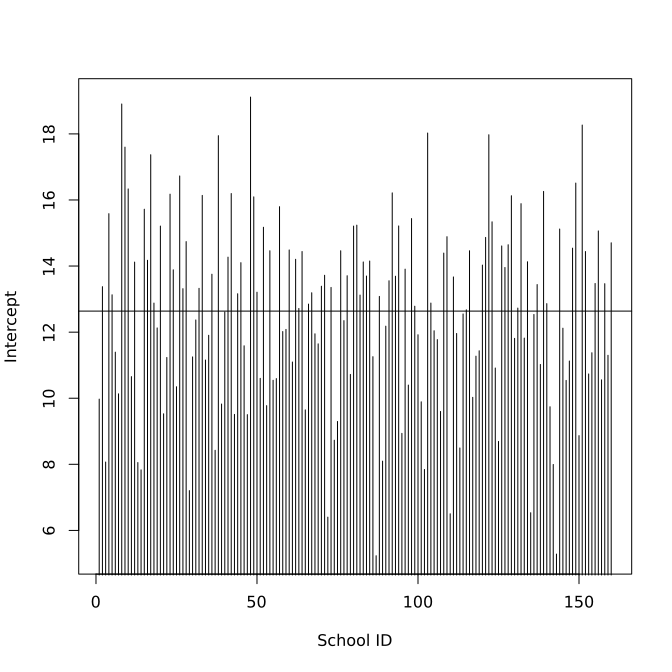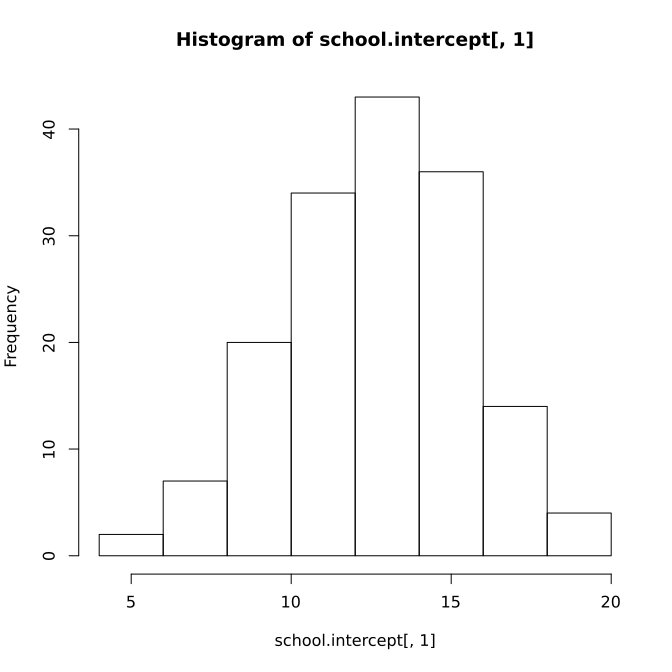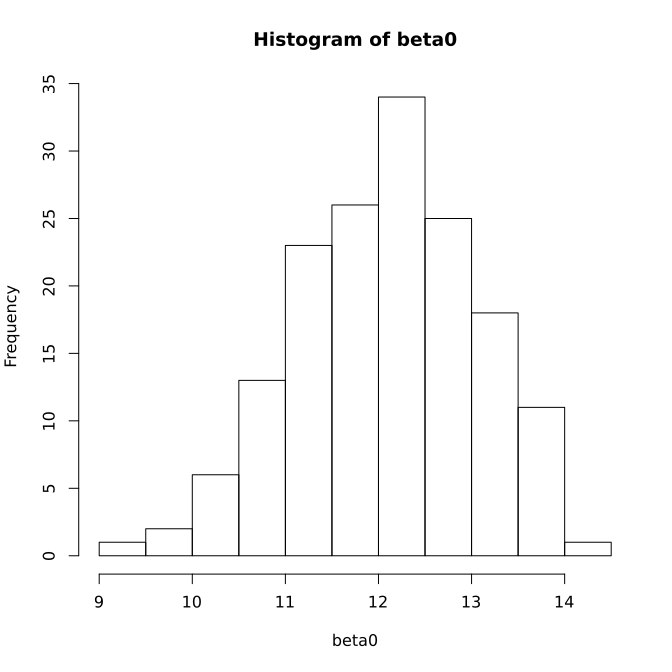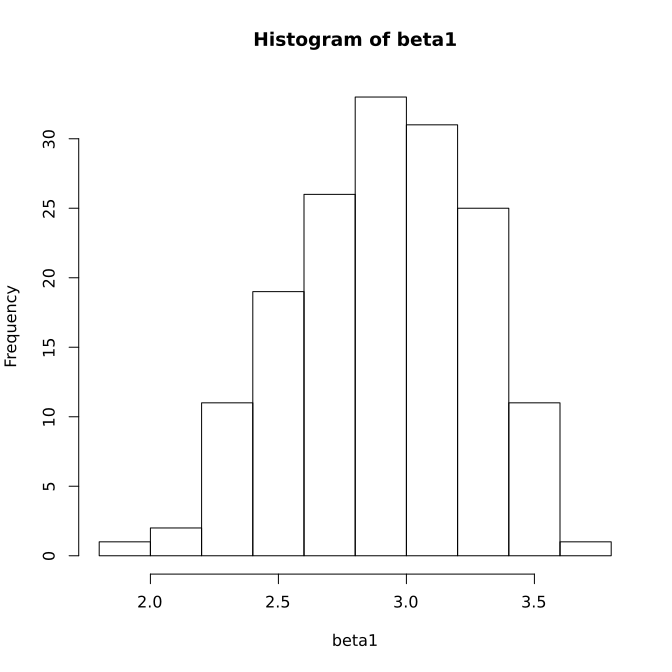# Multilevel Regression

## Multilevel data

Lee and Bryk (1989) analyzed a set of data in illustrating the use of multilevel modeling. The data set includes mathematics scores for senior-year high school students from 160 schools. For each student, information on her/his social and economic status (SES) is also available. For each school, data are available on the sector (private or public) of the schools. In addition, a school level variable called average SES (MSES) was created from the students' SES to measure the SES of each school.

There is a clear two-level structure in the data. At the first level, the student level, we have information on individual students. At the second level, the school level, we have information on schools. The students are nested within schools. This kind of data are called multilevel data.

### Lee and Bryk school achievement data

As an example, we use a subset of data from the Lee and Bryk (1989) study. In total, there are $n=7,185$ students at the first level. At the second level, there are $J=160$ schools. The data are saved in the file LeeBryk.csv. A subset of data are shown below. sector=0 indicates a public school and sector=1 indicates a private Catholic high school.

> usedata('LeeBryk')
schoolid  math   ses  mses sector
1        1  5.88 -1.53 -0.43      0
2        1 19.71 -0.59 -0.43      0
3        1 20.35 -0.53 -0.43      0
4        1  8.78 -0.67 -0.43      0
5        1 17.90 -0.16 -0.43      0
6        1  4.58  0.02 -0.43      0
>


## Source of variances

We first look at the mathematical variable alone. The variance of the variable math is 47.31. The variance can be viewed as the residual variance after removing the mean of it or the residual variance by fitting a regression model with intercept only to it. This assumes that every student across all schools has the same mean score or intercept such that

$math_{ij} = \beta_0 + e_{ij}.$

Given there are 160 schools, it is more reasonable to believe that the intercept (or average math score) is different for each school. Using a regression model way, we then have

$math_{ij}=\beta_{j}+e_{ij},$

where $\beta_j$ is the average math score or intercept for each school $j$.

Because the intercept is different, it can also has its own variation or variance across schools which can also be calculated using a regression model with intercept only

$\beta_{j}=\beta_{0}+v_{j}$

where $v_j$ is the deviation for the average score of school $j$ from the overall average $\beta_0$.

With such a specification, the variance of math can be expressed as the sum of the variance of the residuals $e_{ij}$ -- within-school variance, and the variance of $v_{j}$ -- between-school variance. Specifically, the variance of math is equal to $8.614 + 39.148$ = between-school variance + within-school variance.

Combining the two regressions, we have a two-level regression model. Note that the model can be written as

$math_{ij}=\beta_{0}+v_{j}+e_{ij}.$

The model is called a mixed-effects model in which $\beta_{0}$ is called the fixed effect. It is the average intercept for all schools and $v_{j}$ is called the random effect.

### Use of R package lme4

A multilevel model or a mixed-effects model can be estimated using the R package lme4. Particularly, the function lmer() should be used. The function not only estimates the fixed-effects $\beta_0$ but also the random-effects $v_{j}$. The function use the format lmer(math~1 + (1|schoolid), data=school). In the function, the first "1" tells to estimate a fixed-effects as the overall intercept. (1|schoolid) tells there is a random component in the intercept.

The output includes the Fixed effects, for which a t-test is also conducted for its significance. The Random effects part includes the variance of the residuals ($e_{ij}$) and the variance of the random intercept ($v_j$).

> library(lme4)
> usedata('LeeBryk')
>
> m2<-lmer(math ~ 1 + (1|schoolid), data=LeeBryk)
> summary(m2)
Linear mixed model fit by REML ['lmerMod']
Formula: math ~ 1 + (1 | schoolid)
Data: LeeBryk

REML criterion at convergence: 47116.7

Scaled residuals:
Min       1Q   Median       3Q      Max
-3.06294 -0.75337  0.02658  0.76060  2.74221

Random effects:
Groups   Name        Variance Std.Dev.
schoolid (Intercept)  8.614   2.935
Residual             39.148   6.257
Number of obs: 7185, groups:  schoolid, 160

Fixed effects:
Estimate Std. Error t value
(Intercept)  12.6374     0.2444   51.71
>


The random-effects $v_j$ can be obtained using the function ranef(). With them, the intercept or average math score for each school can be calculated and also plotted as shown below.

> library(lme4)
> usedata('LeeBryk')
>
> m2<-lmer(math ~ 1 + (1|schoolid), data=LeeBryk)
Warning message:
'rBind' is deprecated.
Since R version 3.2.0, base's rbind() should work fine with S4 objects
> bi<-ranef(m2)
>
> school.intercept <- bi$schoolid + 12.6374 > plot(school.intercept[,1],type='h', + xlab='School ID', ylab='Intercept') > abline(h=12.6374) > > hist(school.intercept[,1]) >### Intraclass correlation coefficient (ICC) The intraclass correlation coefficient is defined as the ratio of the variance explained by the multilevel structure and the variance of the outcome variable. For the example above, we have intraclass correlation coefficient $\tau=\frac{8.614}{8.614+39.148}=0.18.$ In social science, it often ranges from 0.05 to 0.25. When ICC is large, it means the between-class variance cannot be ignored and therefore a multilevel model is preferred. It has been suggested that if ICC > 0.1, one should consider the use of a multilevel model. ### Explain/model the difference We have shown the differences in the average score or intercept for each school. What causes the differences? School level covariates, such as the average SES or whether the school is private or public, can be used to explore potential factors related to it. In using a two-level model, we can specify a model as \begin{eqnarray*}math_{ij} & = & \beta_{j}+e_{ij}\\ \beta_{j} & = & \beta_{0}+\beta_{1}mses_{j}+\beta_{2}sector_{j}+v_{j}. \end{eqnarray*} Using a mixed-effect model, it can be written as $math_{ij} = \beta_{0}+\beta_{1}mses_{j}+\beta_{2}sector_{j}+v_{j}+e_{ij},$ where$\beta_k, k=0,1,2$are fixed-effects parameters. Based on the output of the lmer(), both mses and sector are significant given the t-values in the fixed effects table. Note that to get the associated p-value, the R package lmerTest can be used. > library(lme4) Loading required package: Matrix > library(lmerTest) Attaching package: 'lmerTest' The following object is masked from 'package:lme4': lmer The following object is masked from 'package:stats': step > usedata('LeeBryk') > > m3<-lmer(math~1 + mses + sector + (1|schoolid), data=LeeBryk) > summary(m3) Linear mixed model fit by REML t-tests use Satterthwaite approximations to degrees of freedom [lmerMod] Formula: math ~ 1 + mses + sector + (1 | schoolid) Data: LeeBryk REML criterion at convergence: 46946.3 Scaled residuals: Min 1Q Median 3Q Max -3.08323 -0.75079 0.01932 0.76659 2.78831 Random effects: Groups Name Variance Std.Dev. schoolid (Intercept) 2.312 1.520 Residual 39.161 6.258 Number of obs: 7185, groups: schoolid, 160 Fixed effects: Estimate Std. Error df t value Pr(>|t|) (Intercept) 12.0994 0.1986 160.5500 60.919 < 2e-16 *** mses 5.3336 0.3685 151.0000 14.475 < 2e-16 *** sector 1.2196 0.3058 149.6000 3.988 0.000104 *** --- Signif. codes: 0 '***' 0.001 '**' 0.01 '*' 0.05 '.' 0.1 ' ' 1 Correlation of Fixed Effects: (Intr) mses mses 0.246 sector -0.698 -0.357 > > anova(m3) Analysis of Variance Table of type III with Satterthwaite approximation for degrees of freedom Sum Sq Mean Sq NumDF DenDF F.value Pr(>F) mses 8205.1 8205.1 1 151.0 209.522 < 2.2e-16 *** sector 622.9 622.9 1 149.6 15.907 0.0001038 *** --- Signif. codes: 0 '***' 0.001 '**' 0.01 '*' 0.05 '.' 0.1 ' ' 1 >  ## Multilevel regression The variable math can be predicted by certain variables such as individual SES. If we ignore the multilevel structure, we can fit a simple regression as $math_{ij}=\beta_{0}+\beta_{1}ses_{ij}+e_{ij}.$ This is to assume that the relationship, the slope, between math and ses is the same. However, as we showed earlier, the intercepts are different for different schools. So the slopes can also be different. In this case, the model should be written as $math_{ij}=\beta_{0j}+\beta_{1j}ses_{ij}+e_{ij},$ where $\beta_{0j}$ and $\beta_{1j}$ are intercept and slope for the$j$th school. We can also predict the intercept and slope using the school level covariates such as the sector of the school and the SES of the school. Then, we would have a two-level model shown below: \begin{eqnarray*} math_{ij} & = & \beta_{0j}+\beta_{1j}ses_{ij}+e_{ij}\\ \beta_{0j} & = & \gamma_{0}+\gamma_{1}mses_{j}+\gamma_{2}sector_{j}+v_{0j}.\\ \beta_{1j} & = & \gamma_{3}+\gamma_{4}mses_{j}+\gamma_{5}sector_{j}+v_{1j}\end{eqnarray*} The above two-level model can again be written as a mixed-effects model \begin{eqnarray} math_{ij} & = & \beta_{0j}+\beta_{1j}ses_{ij}+e_{ij}\nonumber \\ & = & \gamma_{0}+\gamma_{1}mses_{j}+\gamma_{2}sector_{j}+v_{0j}\nonumber \\ & & +(\gamma_{3}+\gamma_{4}mses_{j}+\gamma_{5}sector_{j}+v_{1j})*ses_{ij}+e_{ij}. \end{eqnarray} To use the R package for model estimation, we first need to plug in the second level equation to the first level to get a mixed model. In the mixed model,$\gamma$s are fixed-effects and$v_{0j}$and$v_{1j}$are random effects. The variances of$v_{0j}$and$v_{1j}$and their correlation can also be obtained. We can also get the variance of$e_{ij}$. Those variance parameters are called random-effects parameters while$\gamma$s are called fixed-effects parameters. In R, each term in the mixed-effects model needs to be specified except for$e_{ij}$. The random effects are specified using the notation | where before it are the random-effects terms and after it is the grouping variable or class variable. For the math example, the R input and output shown below. > library(lme4) Loading required package: Matrix > library(lmerTest) Attaching package: 'lmerTest' The following object is masked from 'package:lme4': lmer The following object is masked from 'package:stats': step > usedata('LeeBryk') > > m4<-lmer(math~1 + mses + sector + + ses + ses*mses + ses*sector + + (1 + ses|schoolid), data=LeeBryk) > summary(m4) Linear mixed model fit by REML t-tests use Satterthwaite approximations to degrees of freedom [lmerMod] Formula: math ~ 1 + mses + sector + ses + ses * mses + ses * sector + (1 + ses | schoolid) Data: LeeBryk REML criterion at convergence: 46505.1 Scaled residuals: Min 1Q Median 3Q Max -3.14225 -0.72479 0.01375 0.75506 2.98329 Random effects: Groups Name Variance Std.Dev. Corr schoolid (Intercept) 2.40703 1.5515 ses 0.01443 0.1201 1.00 Residual 36.75766 6.0628 Number of obs: 7185, groups: schoolid, 160 Fixed effects: Estimate Std. Error df t value Pr(>|t|) (Intercept) 12.1026 0.2028 166.0000 59.678 < 2e-16 *** mses 3.3225 0.3885 178.0000 8.553 5.33e-15 *** sector 1.1882 0.3081 148.0000 3.857 0.000171 *** ses 2.9057 0.1483 4352.0000 19.595 < 2e-16 *** mses:ses 0.8476 0.2717 3546.0000 3.119 0.001829 ** sector:ses -1.5793 0.2246 4347.0000 -7.033 2.34e-12 *** --- Signif. codes: 0 '***' 0.001 '**' 0.01 '*' 0.05 '.' 0.1 ' ' 1 Correlation of Fixed Effects: (Intr) mses sector ses mss:ss mses 0.213 sector -0.676 -0.345 ses 0.077 -0.146 -0.065 mses:ses -0.143 0.179 -0.082 0.279 sector:ses -0.062 -0.081 0.094 -0.679 -0.357 > anova(m4) Analysis of Variance Table of type III with Satterthwaite approximation for degrees of freedom Sum Sq Mean Sq NumDF DenDF F.value Pr(>F) mses 2688.9 2688.9 1 178.5 73.15 5.329e-15 *** sector 546.8 546.8 1 148.3 14.88 0.0001707 *** ses 14113.4 14113.4 1 4351.7 383.96 < 2.2e-16 *** mses:ses 357.6 357.6 1 3546.5 9.73 0.0018288 ** sector:ses 1818.0 1818.0 1 4346.7 49.46 2.342e-12 *** --- Signif. codes: 0 '***' 0.001 '**' 0.01 '*' 0.05 '.' 0.1 ' ' 1 >  The parameters in the table of Fixed effects give the estimates for$\gamma$s. Hence, we have \begin{eqnarray*} \beta_{0j} & = & 12.10+3.32mses_{j}+1.19sector_{j}+v_{0j}\\ \beta_{1j} & = & 2.91+0.85mses_{j}-1.58sector_{j}+v_{1j} \end{eqnarray*} Note that based on the F-test, both ses and the sector of the school are significant predictors of both intercept and slope. The parameters in the table of Random effects give the variance estimates. Therefore, the variance of$e_{ij}$is 36.76. The variance of$v_{0j}$is 2.41 and the variance of$v_{1j}$is .014. The correlation between$v_{0j}$and$v_{1j}$is almost 1. Individual values for$v_{0j}$and$v_{1j}$can be obtained using the function ranef(). Note that with the fixed effects and random effects, we can calculate the intercept$\beta_{0j}$and slope$\beta_{1j}$for each school. Then, the individual regression line can be plotted together with the scatterplot of all data. > library(lme4) Loading required package: Matrix > library(lmerTest) Attaching package: 'lmerTest' The following object is masked from 'package:lme4': lmer The following object is masked from 'package:stats': step > usedata('LeeBryk') > attach(LeeBryk) > > m4<-lmer(math~1 + mses + sector + + ses + ses*mses + ses*sector + + (1 + ses|schoolid), data=LeeBryk) Warning message: 'rBind' is deprecated. Since R version 3.2.0, base's rbind() should work fine with S4 objects > > ## calculate betas > #random effects > rancof<-ranef(m4)$schoolid
> beta0<-beta1<-rep(0, 160)
>
> for (i in 1:160){
+   index<-min(which(schoolid==i))
+   beta0[i] <- 12.1026+2.3225*mses[index]
+               +1.1882*sector[index]+rancof[i,1]
+   beta1[i] <- 2.9057+0.8476*mses[index]
+               -1.5793*sector[index]+rancof[i,2]
+ }
>
> hist(beta0)
> hist(beta1)
>
> ## plot the relationship for each school
> plot(ses, math)
>
> for (i in 1:160){
+   abline(beta0[i], beta1[i])
+ }
>